1:29 AM Dynamics of Motion in a Central Potential: Deriving Kepler's Laws Dynamics of Motion in a Central Potential: Deriving Kepler's Laws Conserved Quantities The equation of motion is: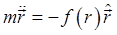. Here we use the hat ^ to denote a unit vector, so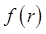gives the magnitude (and sign) of the force. For Kepler's problem,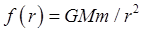. (Strictly speaking, we should be using the reduced mass for planetary motion, for our Solar System, that is a small correction. It can be put in at the end if needed.) Let's see how using vector methods we can easily find constants of motion: first, angular momentum -- just act on the equation of motion with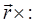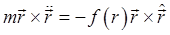Since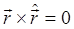, we have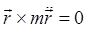, which immediately integrates to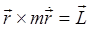, a constant, the angular momentum, and note that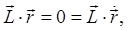so the motion will always stay in a plane, with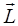perpendicular to the plane. This establishes that motion in a purely central force obeys a conservation law: that of angular momentum. (As we've discussed earlier in the course, conserved quantities in dynamical systems are always related to some underlying symmetry of the Hamiltonian. The conservation of angular momentum comes from the spherical symmetry of the system: the attraction depends only on distance, not angle. In quantum mechanics, the angular momentum operator is a rotation operator: the three components of the angular momentum vector are conserved, are constants of the motion, because the Hamiltonian is invariant under rotation. That is, the angular momentum operators commute with the Hamiltonian. The classical analogy is that they have zero Poisson brackets with the Hamiltonian.) To get back to Kepler's statement of his Laws, notice that when the planet moves through an incremental distance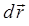it "sweeps out" an area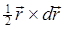, so the rate of sweeping out area is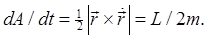Kepler's Second Law is just conservation of angular momentum! Second, conservation of energy: this time, we act on the equation of motion with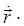: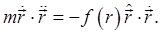This immediately integrates to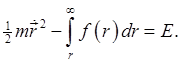Another conservation law coming from a simple integral: conservation of energy. What symmetry does thatcorrespond to? The answer is the invariance of the Hamiltonian under time: the central force is time invariant, and we're assuming there are time-dependent potential terms, (such as from another star passing close by). Standard Calculus Derivation of Kepler's First Law The first mathematical proof that an elliptic orbit about a focus meant an inverse-square attraction was given by Newton, using Euclidean geometry (even though he invented calculus!). The proof is notoriously difficult to follow. Bernoulli found a fairly straightforward calculus proof in polar coordinates by changing the variable to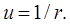The first task is to express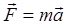in polar, meaning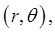coordinates. The simplest way to find the expression for acceleration is to parameterize the planar motion as a complex number: position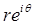, velocity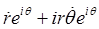, notice this means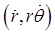since theensures the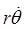term is in the positive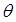direction, and differentiating again gives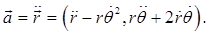For a central force, the only acceleration is in the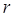direction, so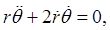which integrates to give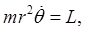the constancy of angular momentum. Equating the radial components,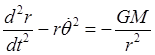which isn't ready to integrate yet, because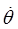varies too. But since the angular momentum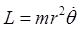is constant, we can eliminatefrom the equation, giving: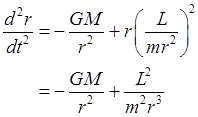This doesn't look too promising, but Bernoulli came up with two clever tricks. The first was to change from the variableto its inverse,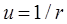. The other was to use the constancy of angular momentum to change the variableto. Putting these together: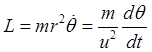so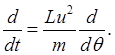Therefore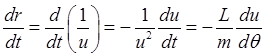and similarly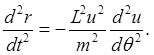Going fromto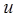in the equation of motion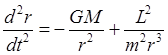we get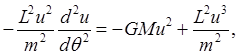or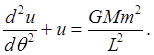This equation is easy to solve! The solution is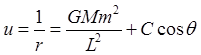where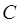is a constant of integration, determined by the initial conditions. This proves that Kepler's First Law follows from the inverse-square nature of the force, because (see beginning of lecture) the equation above is exactly the standard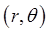equation of an ellipse of semi major axis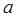and eccentricity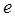, with the origin at one focus: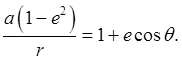Comparing the two equations, we can find the geometry of the ellipse in terms of the angular momentum, the gravitational attraction, and the initial conditions. The angular momentum is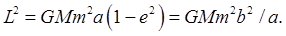.u-star-rating-16 { list-style:none; margin:0px; padding:0px; width:80px; height:16px; position:relative; background: url('/.s/t/1211/rating.png') top left repeat-x } .u-star-rating-16 li{ padding:0px; margin:0px; float:left } .u-star-rating-16 li a { display:block;width:16px;height: 16px;line-height:16px;text-decoration:none;text-indent:-9000px;z-index:20;position:absolute;padding: 0px;overflow:hidden } .u-star-rating-16 li a:hover { background: url('/.s/t/1211/rating.png') left center;z-index:2;left:0px;border:none } .u-star-rating-16 a.u-one-star { left:0px } .u-star-rating-16 a.u-one-star:hover { width:16px } .u-star-rating-16 a.u-two-stars { left:16px } .u-star-rating-16 a.u-two-stars:hover { width:32px } .u-star-rating-16 a.u-three-stars { left:32px } .u-star-rating-16 a.u-three-stars:hover { width:48px } .u-star-rating-16 a.u-four-stars { left:48px } .u-star-rating-16 a.u-four-stars:hover { width:64px } .u-star-rating-16 a.u-five-stars { left:64px } .u-star-rating-16 a.u-five-stars:hover { width:80px } .u-star-rating-16 li.u-current-rating { top:0 !important; left:0 !important;margin:0 !important;padding:0 !important;outline:none;background: url('/.s/t/1211/rating.png') left bottom;position: absolute;height:16px !important;line-height:16px !important;display:block;text-indent:-9000px;z-index:1 } Category: Education | Views: 318 | Added by: farrel | | Rating: 0.0/0